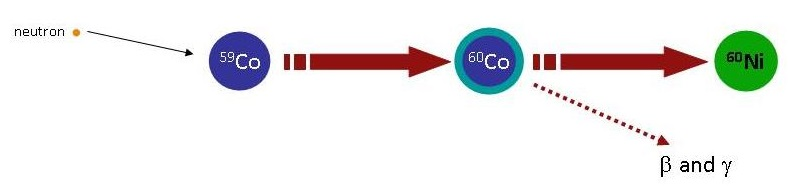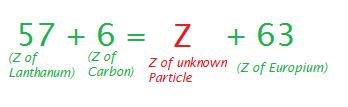$$\newcommand{\vecs}{\overset { \rightharpoonup} {\mathbf{#1}} }$$ $$\newcommand{\vecd}{\overset{-\!-\!\rightharpoonup}{\vphantom{a}\smash {#1}}}$$$$\newcommand{\id}{\mathrm{id}}$$ $$\newcommand{\Span}{\mathrm{span}}$$ $$\newcommand{\kernel}{\mathrm{null}\,}$$ $$\newcommand{\range}{\mathrm{range}\,}$$ $$\newcommand{\RealPart}{\mathrm{Re}}$$ $$\newcommand{\ImaginaryPart}{\mathrm{Im}}$$ $$\newcommand{\Argument}{\mathrm{Arg}}$$ $$\newcommand{\norm}{\| #1 \|}$$ $$\newcommand{\inner}{\langle #1, #2 \rangle}$$ $$\newcommand{\Span}{\mathrm{span}}$$ $$\newcommand{\id}{\mathrm{id}}$$ $$\newcommand{\Span}{\mathrm{span}}$$ $$\newcommand{\kernel}{\mathrm{null}\,}$$ $$\newcommand{\range}{\mathrm{range}\,}$$ $$\newcommand{\RealPart}{\mathrm{Re}}$$ $$\newcommand{\ImaginaryPart}{\mathrm{Im}}$$ $$\newcommand{\Argument}{\mathrm{Arg}}$$ $$\newcommand{\norm}{\| #1 \|}$$ $$\newcommand{\inner}{\langle #1, #2 \rangle}$$ $$\newcommand{\Span}{\mathrm{span}}$$$$\newcommand{\AA}{\unicode[.8,0]{x212B}}$$

Radioactivity is the process by which the nucleus of an unstable atom loses energy by emitting radiation, including alpha particles, beta particles, gamma rays and conversion electrons Although radioactivity is observed as a natural occurring process, it can also be artificially induced typically via the bombarding atoms of a specific element by radiating particles, thus creating new atoms.

## Introduction

Ernest Rutherford was a prominent New Zealand scientist, and a winner of the Nobel Prize in chemistry in 1908. Amongst his vast list of discoveries, Rutherford was also the first to discover artificially induced radioactivity. Through the bombardment of alpha particles against nuclei of $$\ce{^{14}N}$$ with 7 protons/electrons, Rutherford produced $$\ce{^{17}O}$$ (8 protons/electrons) and protons (Figure $$\PageIndex{1}$$). Through this observation, Rutherford concluded that atoms of one specific element can be made into atoms of another element. If the resulting element is radioactive, then this process is called artificially induced radioactivityFigure $$\PageIndex{1}$$: Rutherford generated $$\ce{^{17}O}$$ via bombarding $$\ce{^{14}N}$$ with alpha particles.

Rutherford was the first researcher to create protons outside of the atomic nuclei and the $$\ce{^{17}O}$$ isotope of oxygen, which is nonradioactive. Similarly, other nuclei when bombarded with alpha particles will generate new elements (Figure $$\PageIndex{2}$$) that may be radioactive and decay naturally or that may be stable and persist like $$\ce{^{17}O}$$.Figure $$\PageIndex{2}$$: Researchers have used $$\alpha$$ particle to react with another atom such as Beryllium. The result is a Carbon nucleus and a neutron. This is artificial radioactivity or induced radioactivity. from igem.org.

Before this discovery of artificial induction of radioactivity, it was a common belief that atoms of matter are unchangeable and indivisible. After the very first discoveries made by Ernest Rutherford, Irene Joliot-Curie and her husband, Frederic Joliot, a new point of view was developed. The point of view that although atoms appear to be stable, they can be transformed into new atoms with different chemical properties. Today over one thousand artificially created radioactive nuclides exist, which considerably outnumber the nonradioactive ones created.

##### Note: Irene Joliet-Curie and Frederic Joliot

Irene Joliet-Curie and her husband Frédéric both were French scientists who shared winning the Nobel Prize award in chemistry in 1935 for artificially synthesizing a radioactive isotope of phosphorus by bombarding aluminum with alpha particles. $$\ce{^{30}P}$$ with 15 protons was the first radioactive nuclide obtained through this method of artificially inducing radioactivity.

$\ce{^27_13Al + ^4_2He \rightarrow ^30_15P + ^1_0n}$

$\ce{^30_15P \rightarrow ^30_14Si + ^0_{-1}\beta}$Figure $$\PageIndex{3}$$: Researchers can use neutrons to react with atoms (for example 235 Uranium) to generate a bigger nuclei with an excess of neutron leading to an increase of the unstability and the new nucleus can split into two smaller nuclei. This phenomenon is the sometimes called neutronic fission. from igem.org.

Activation (or radioactivation) involves making a radioactive isotope by neutron capture, e.g. the addition of a neutron to a nuclide resulting in an increase in isotope number by 1 while retaining the same atomic number (Figure $$\PageIndex{3}$$). Activation is often an inadvertent process occurring inside or near a nuclear reactor, where there are many neutrons flying around. For example, Coba in or near a nuclear reactor will capture a neutron forming the radioactive isotope Co-60.

$\ce{ ^1_0n + ^{59}Co \rightarrow ^{60}Co }$

The $$\ce{ ^{60}Co}$$ isotope is unstable (half life of 5.272 years) and disintegrates into $$\ce{ ^{60}Ni }$$ via the emission of $$\beta$$ particle and $$\gamma$$ radiation Figure $$\PageIndex{4}$$.Figure $$\PageIndex{4}$$: Example of $$\ce{ ^{59}Co }$$ activation and $$\ce{ ^{60}Co }$$ disintegration. from igem.org.
##### Example $$\PageIndex{1}$$: Neutron Bombardment

Write a nuclear equation for the creation of 56Mn through the bombardment of 59Co with neutrons.

Solution

A unknown particle is produced with 56Mn, in order to find the mass number (A) of the unknown we must subtract the mass number of the Manganese atom from the mass number of the Cobalt atom plus the neutron being thrown. In simpler terms,Now, by referring to a periodic table to find the atomic numbers of Mn and Co, and then subtracting the atomic number of Mn from Co, we will receive the atomic number of the unknown particleThus, the unknown particle has A = 4, and Z = 2, which would make it a Helium particle, and the nuclear formula would be as follows:

$\ce{^{50}_{27}Co + ^1_0n \rightarrow ^{56}_{25}Mn + ^{4}_{2}\alpha } \nonumber$

##### Example $$\PageIndex{2}$$: Calcium Bombardment

Write a nuclear equation for the production of $$\ce{^{147}Eu}$$ by bombardment of $$\ce{^{139}La}$$ with $$\ce{^{12}Ca}$$.

Solution

Like the above example, you must first find the mass number of the unknown particle.Thus, the mass number of the unknown particle is 4. Again by referring to a periodic table and finding the atomic numbers of Lanthanum, Carbon and Europium, we are able to calculate the atomic number of the unknown particle,The atomic number for the unknown particle equals to zero, therefore 4 neutrons are emitted, and the nuclear equation is written as follows:

$\ce{^{139}_{57}La + ^{12}_6C \rightarrow ^{147}_{63}Eu + 4 ^{0}_{1}n } \nonumber$